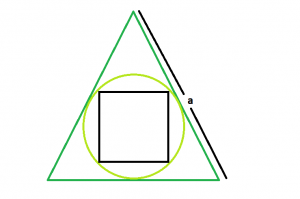# Area of a square inscribed in a circle which is inscribed in an equilateral triangle

• Last Updated : 15 Mar, 2021

Given here is an equilateral triangle with side length a, which inscribes a circle, which in turn inscribes a square. The task is to find the area of this square.
Examples:

```Input: a = 6
Output: 1

Input: a = 10
Output: 0.527046```

Attention reader! Don’t stop learning now. Get hold of all the important DSA concepts with the DSA Self Paced Course at a student-friendly price and become industry ready.  To complete your preparation from learning a language to DS Algo and many more,  please refer Complete Interview Preparation Course.

In case you wish to attend live classes with experts, please refer DSA Live Classes for Working Professionals and Competitive Programming Live for Students.Approach:

let r be the radius of circle,
hence it is the inradius of equilateral triangle, so r = a /(2 * √3)
diagonal of square, d = diameter of circle = 2 * r = a/ √3
So, area of square, A = 0.5 * d * d
hence A = (1/2) * (a^2) / (3) = (a^2/6)

Below is the implementation of the above approach:

## C++

 `// C++ Program to find the area of the square``// inscribed within the circle which in turn``// is inscribed in an equilateral triangle``#include ``using` `namespace` `std;` `// Function to find the area of the square``float` `area(``float` `a)``{` `    ``// a cannot be negative``    ``if` `(a < 0)``        ``return` `-1;` `    ``// area of the square``    ``float` `area = ``sqrt``(a) / 6;` `    ``return` `area;``}` `// Driver code``int` `main()``{``    ``float` `a = 10;``    ``cout << area(a) << endl;``    ``return` `0;``}`

## Java

 `// Java Program to find the area of the square``// inscribed within the circle which in turn``// is inscribed in an equilateral triangle` `import` `java.io.*;` `class` `GFG {``   `  `// Function to find the area of the square``static` `float` `area(``float` `a)``{` `    ``// a cannot be negative``    ``if` `(a < ``0``)``        ``return` `-``1``;` `    ``// area of the square``    ``float` `area = (``float``)Math.sqrt(a) / ``6``;` `    ``return` `area;``}` `// Driver code``    ``public` `static` `void` `main (String[] args) {``        ``float` `a = ``10``;``    ``System.out.println( area(a));``// This code is contributed``// by  inder_verma..``    ``}``}`

## Python 3

 `# Python3 Program to find the area``# of the square inscribed within ``# the circle which in turn is``# inscribed in an equilateral triangle` `# import everything from math lib.``from` `math ``import` `*` `# Function to find the area``# of the square``def` `area(a):` `    ``# a cannot be negative``    ``if` `a < ``0` `:``        ``return` `-``1` `    ``# area of the square``    ``area ``=` `sqrt(a) ``/` `6` `    ``return` `area` `# Driver code    ``if` `__name__ ``=``=` `"__main__"` `:` `    ``a ``=` `10``    ``print``(``round``(area(a), ``6``))` `# This code is contributed by ANKITRAI1`

## C#

 `// C# Program to find the area``// of the square inscribed within``// the circle which in turn is``// inscribed in an equilateral triangle``using` `System;` `class` `GFG``{``    ` `// Function to find the area``// of the square``static` `float` `area(``float` `a)``{` `    ``// a cannot be negative``    ``if` `(a < 0)``        ``return` `-1;` `    ``// area of the square``    ``float` `area = (``float``)Math.Sqrt(a) / 6;` `    ``return` `area;``}` `// Driver code``public` `static` `void` `Main ()``{``    ``float` `a = 10;``    ``Console.WriteLine(area(a));``}``}` `// This code is contributed``// by inder_verma`

## PHP

 ``

## Javascript

 ``
Output:
`0.527046`

My Personal Notes arrow_drop_up